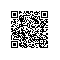# 零值比较--BOOL，int，float，指针变量与零值比较的if语句

if ( n == 0 )

if ( n != 0 )

if ( n )              // 会让人误解 n 是布尔变量

if ( !n  )

if ( flag )

if ( !flag )

if (flag == TRUE)

if (flag == 1 )

if (flag == FALSE)

if (flag == 0)

if (x == 0.0)         // 隐含错误的比较

if ((x>=-EPSINON) && (x<=EPSINON))

const float EPSINON = 0.00001;

if ((x >= - EPSINON) && (x <= EPSINON)

if (x == 0.0)

if (x != 0.0)

if (p == NULL)

if (p != NULL)

if (p == 0)        // 容易让人误解p是整型变量

if (p != 0)

if (p)                // 容易让人误解p是bool型变量

if (!p)

if ((x >= - EPSINON) && (x <= EPSINON) 这样判断是可取的

#define   E   1.0E-6

float   x   与“零值”比较的if
-----------------------

#define   E   0.000001

fabs((a+b)+c)   -   ((b+a)+c))使用钉钉扫一扫加入圈子
+ 订阅